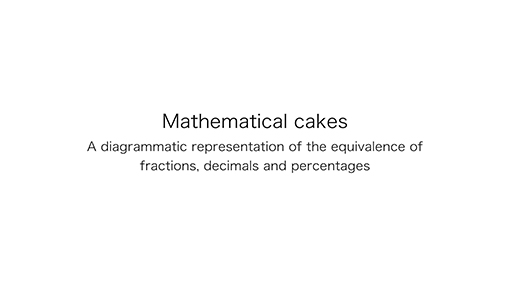Teaching mathematics

Start this free course now. Just create an account and sign in. Enrol and complete the course for a free statement of participation or digital badge if available.

Free course

# 1.1 Diagrammatic representations of equivalence

The first video shows a mathematical cake which is shaped like a rectangle and has been cut into ten slices. Learners can draw their own mathematical cake on paper. It can have a varied length but it is useful to ensure that the width is 10 cm, which means each slice is 1 cm wide. Diagrams can be very useful aids to teaching concepts in mathematics.

Download this video clip.Video player: Video 1 Mathematical cake: a diagrammatic representation of the equivalence of fractions, decimals and percentagesSkip transcript: Video 1 Mathematical cake: a diagrammatic representation of the equivalence of fractions, decimals and percentages

#### Transcript: Video 1 Mathematical cake: a diagrammatic representation of the equivalence of fractions, decimals and percentages

INSTRUCTOR:
Mathematical cakes, a diagrammatic representation of the equivalence of fractions, decimals, and percentages. Here is a mathematical cake, which is rectangular in shape and split into 10 equal slices. We'll consider the percentages first the whole cake is 100%. so one slice must be 100% divided by 10, or 10%. No slices is 0%, one slice is 10%, two slices are 20%, three slices of 30%, and so on and one whole cake is 100%.
Next the decimals, the whole cake is 1.0. So each slice must be 1.0 divided by 10, which is 0.1. No slices is 0.0, one slice is 0.1. two slices are 0.2, three slices are 0.3, and so on. And the whole cake is 1.0.
Finally, the fractions, the whole cake is one whole, so each slice must be 1/10. No slices is 0, one slice is 1/10, two slices are 2/10, three slices are 3/10, and so on. And the whole cake is one whole.
Some of these fractions have equivalent simpler fractions. 5/10 is half of the cake. And that can be seen to be equivalent to 0.5 and 50%. 3/10 is 0.3 and 30%. and 60% is equivalent to 0.6 and 6/10, which can be simplified to 3/5.
You can easily read across to find equivalences from the diagram. The fractions, decimals, and percentages shown on the diagram are some of the important equivalences to know. Supposing you wanted to find out 1/4 from this diagram, how would you find where 1/4 is and work out its equivalences in decimals and percentages? What would you do about 3/4?
End transcript: Video 1 Mathematical cake: a diagrammatic representation of the equivalence of fractions, decimals and percentages
Video 1 Mathematical cake: a diagrammatic representation of the equivalence of fractions, decimals and percentages
Interactive feature not available in single page view (see it in standard view).

## Activity 1 Finding equivalences to one-quarter and three-quarters

Timing: Allow 5 minutes

Look at the mathematical cake diagram which was shown in the video. Use it to find equivalences to and .

### Discussion

The mathematical cake diagram can be used to demonstrate the equivalences of percentages, decimals and fractions in tenths.

One-quarter can be found by finding the mid-way point between 0 and one-half. Three quarters would be found mid-way between one-half and one-whole of the cake.

One-quarter is 25% and 0.25.

Three-quarters is 75% and 0.75.

TM_1